# Copyright 2007 Pearson Education Inc Publishing as Pearson

• Slides: 38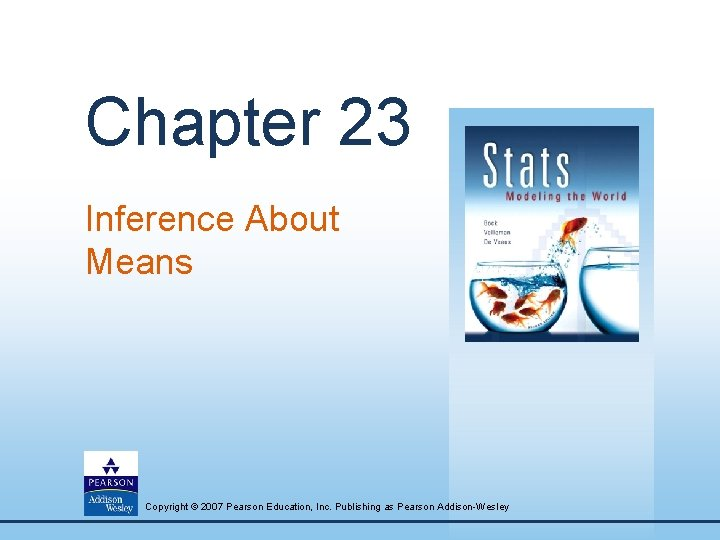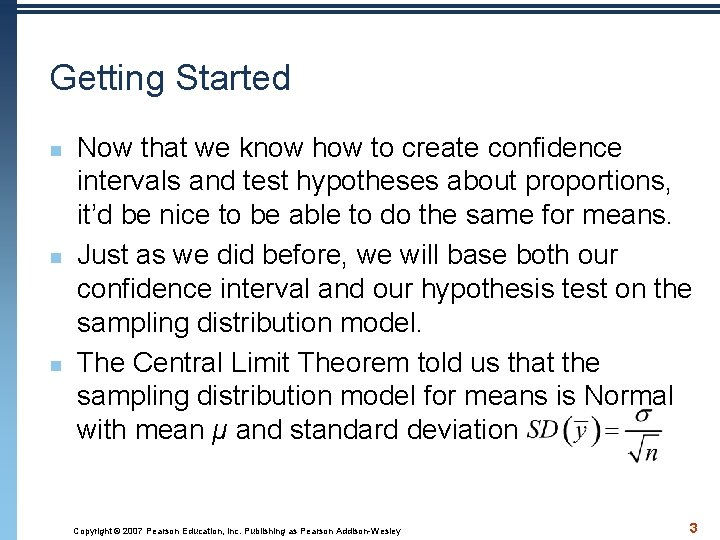Getting Started n n n Now that we know how to create confidence intervals and test hypotheses about proportions, it’d be nice to be able to do the same for means. Just as we did before, we will base both our confidence interval and our hypothesis test on the sampling distribution model. The Central Limit Theorem told us that the sampling distribution model for means is Normal with mean μ and standard deviation Copyright © 2007 Pearson Education, Inc. Publishing as Pearson Addison-Wesley 3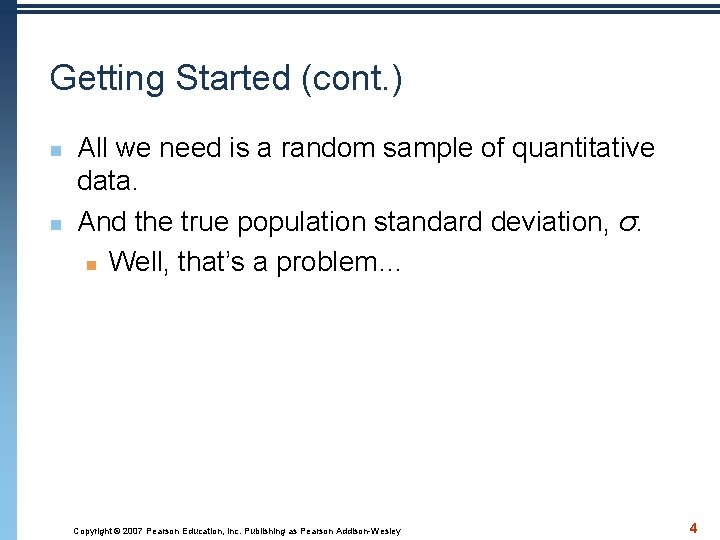Getting Started (cont. ) n n All we need is a random sample of quantitative data. And the true population standard deviation, σ. n Well, that’s a problem… Copyright © 2007 Pearson Education, Inc. Publishing as Pearson Addison-Wesley 4Getting Started (cont. ) n n Proportions have a link between the proportion value and the standard deviation of the sample proportion. This is not the case with means—knowing the sample mean tells us nothing about We’ll do the best we can: estimate the population parameter σ with the sample statistic s. Our resulting standard error is Copyright © 2007 Pearson Education, Inc. Publishing as Pearson Addison-Wesley 5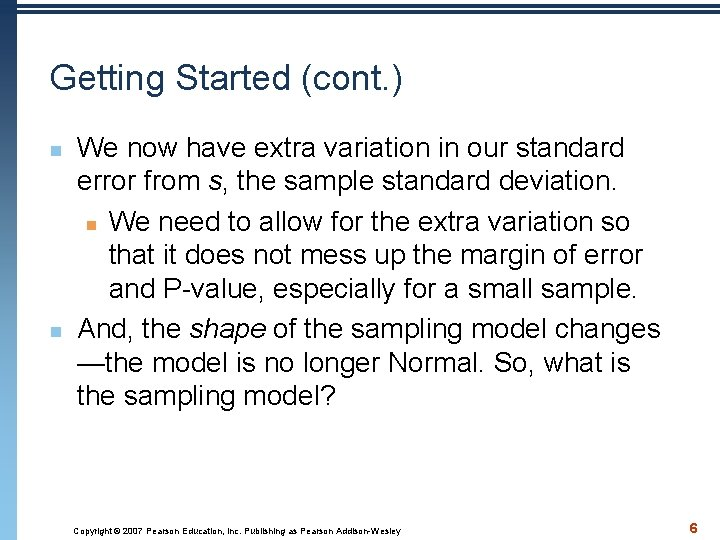Getting Started (cont. ) n n We now have extra variation in our standard error from s, the sample standard deviation. n We need to allow for the extra variation so that it does not mess up the margin of error and P-value, especially for a small sample. And, the shape of the sampling model changes —the model is no longer Normal. So, what is the sampling model? Copyright © 2007 Pearson Education, Inc. Publishing as Pearson Addison-Wesley 6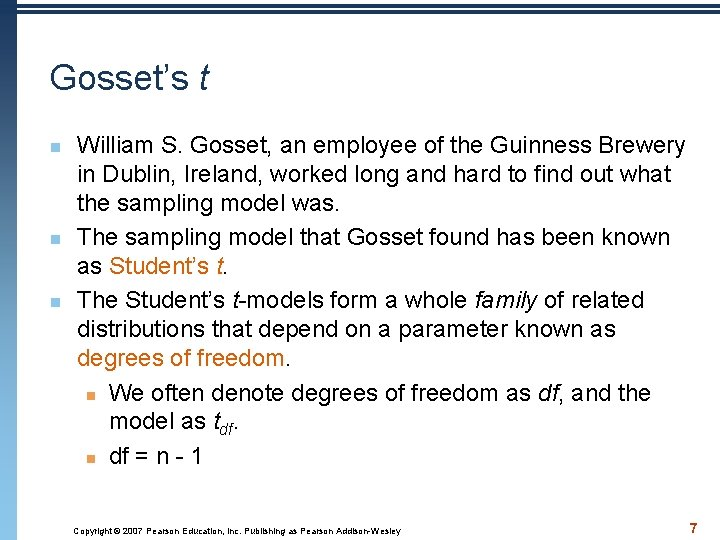Gosset’s t n n n William S. Gosset, an employee of the Guinness Brewery in Dublin, Ireland, worked long and hard to find out what the sampling model was. The sampling model that Gosset found has been known as Student’s t. The Student’s t-models form a whole family of related distributions that depend on a parameter known as degrees of freedom. n We often denote degrees of freedom as df, and the model as tdf. n df = n - 1 Copyright © 2007 Pearson Education, Inc. Publishing as Pearson Addison-Wesley 7What Does This Mean for Means? A practical sampling distribution model for means When the conditions are met, the standardized sample mean follows a Student’s t-model with n – 1 degrees of freedom. We estimate the standard error with Copyright © 2007 Pearson Education, Inc. Publishing as Pearson Addison-Wesley 8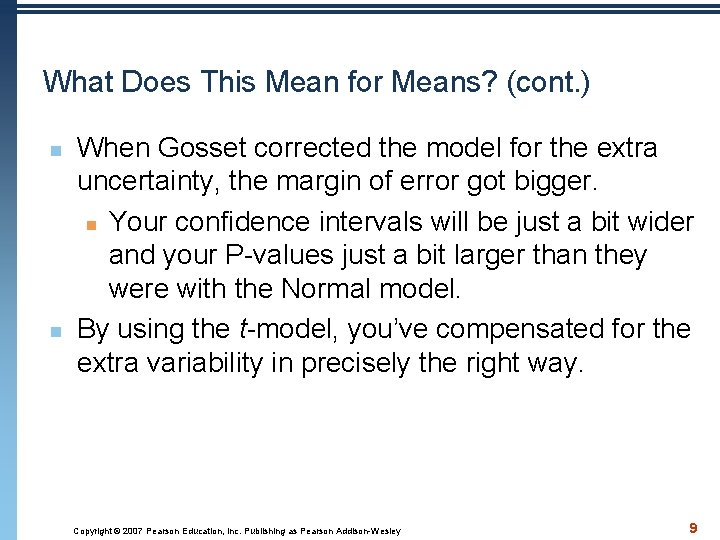What Does This Mean for Means? (cont. ) n n When Gosset corrected the model for the extra uncertainty, the margin of error got bigger. n Your confidence intervals will be just a bit wider and your P-values just a bit larger than they were with the Normal model. By using the t-model, you’ve compensated for the extra variability in precisely the right way. Copyright © 2007 Pearson Education, Inc. Publishing as Pearson Addison-Wesley 9What Does This Mean for Means? (cont. ) n n Student’s t-models are unimodal, symmetric, and bell shaped, just like the Normal. But t-models with only a few degrees of freedom have much fatter tails than the Normal. Copyright © 2007 Pearson Education, Inc. Publishing as Pearson Addison-Wesley 10What Does This Mean for Means? (cont. ) n n As the degrees of freedom increase, the t-models look more and more like the Normal. In fact, the t-model with infinite degrees of freedom is exactly Normal. Copyright © 2007 Pearson Education, Inc. Publishing as Pearson Addison-Wesley 11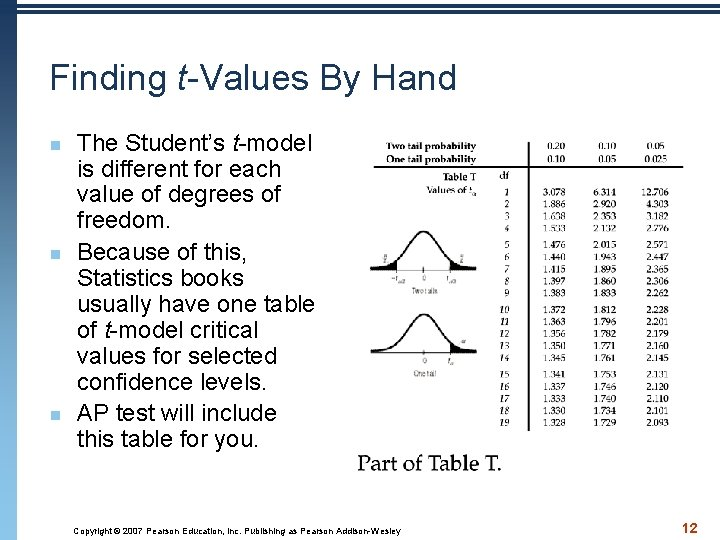Finding t-Values By Hand n n n The Student’s t-model is different for each value of degrees of freedom. Because of this, Statistics books usually have one table of t-model critical values for selected confidence levels. AP test will include this table for you. Copyright © 2007 Pearson Education, Inc. Publishing as Pearson Addison-Wesley 12Finding t values with Calculator inv. T(area from left, degrees of freedom) n n n Area must be a decimal Area must reflect confidence level df is degrees of freedom (df = n – 1) Practice: Find t value for a 95% confidence interval with 17 degrees of freedom. Copyright © 2007 Pearson Education, Inc. Publishing as Pearson Addison-Wesley 13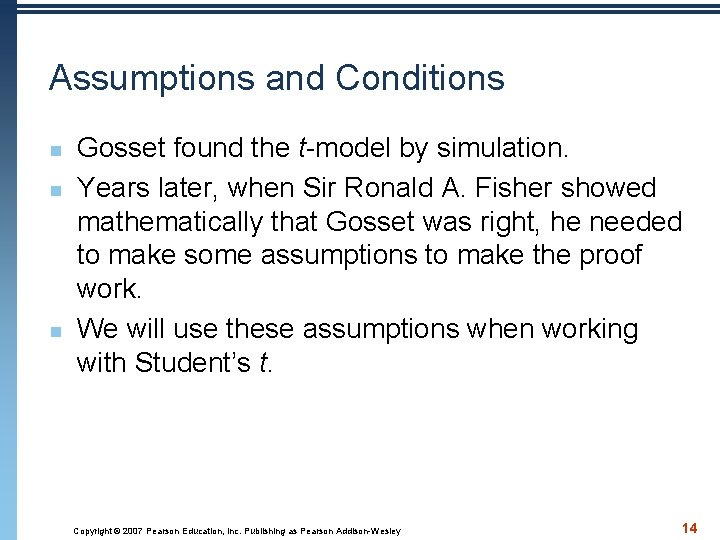Assumptions and Conditions n n n Gosset found the t-model by simulation. Years later, when Sir Ronald A. Fisher showed mathematically that Gosset was right, he needed to make some assumptions to make the proof work. We will use these assumptions when working with Student’s t. Copyright © 2007 Pearson Education, Inc. Publishing as Pearson Addison-Wesley 14Assumptions and Conditions (cont. ) n Independence Assumption: n Randomization Condition: The data arise from a random sample or suitably randomized experiment. Randomly sampled data (particularly from an SRS) are ideal. n 10% Condition: When a sample is drawn without replacement, the sample should be no more than 10% of the population. Copyright © 2007 Pearson Education, Inc. Publishing as Pearson Addison-Wesley 15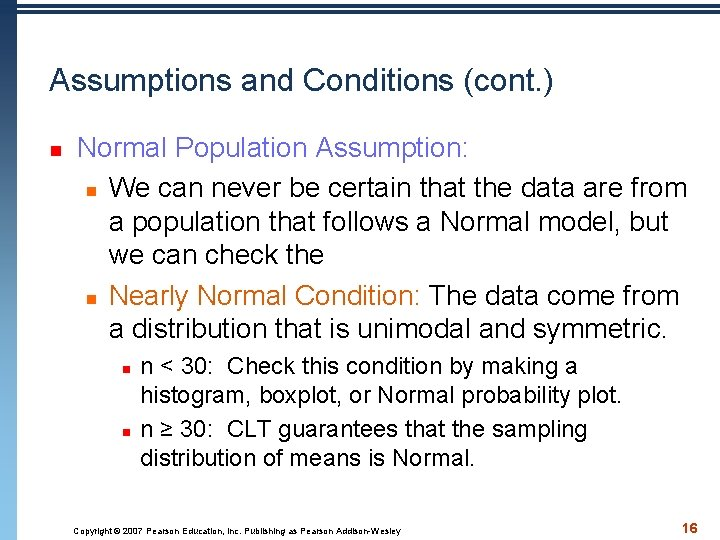Assumptions and Conditions (cont. ) n Normal Population Assumption: n We can never be certain that the data are from a population that follows a Normal model, but we can check the n Nearly Normal Condition: The data come from a distribution that is unimodal and symmetric. n n n < 30: Check this condition by making a histogram, boxplot, or Normal probability plot. n ≥ 30: CLT guarantees that the sampling distribution of means is Normal. Copyright © 2007 Pearson Education, Inc. Publishing as Pearson Addison-Wesley 16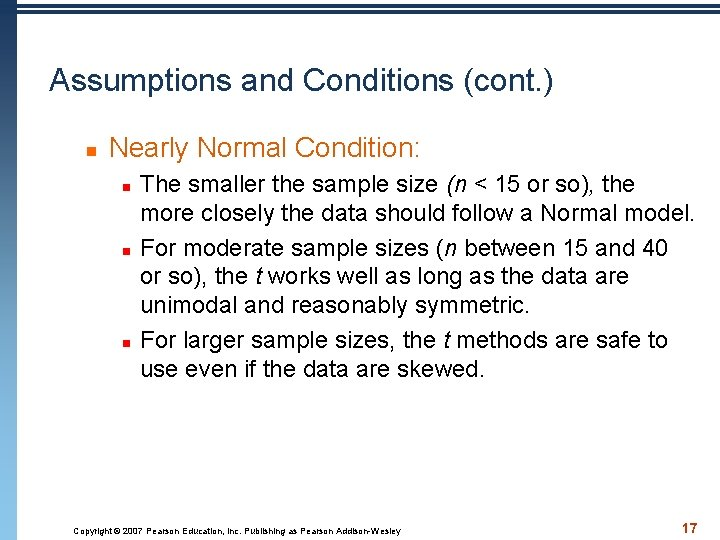Assumptions and Conditions (cont. ) n Nearly Normal Condition: n n n The smaller the sample size (n < 15 or so), the more closely the data should follow a Normal model. For moderate sample sizes (n between 15 and 40 or so), the t works well as long as the data are unimodal and reasonably symmetric. For larger sample sizes, the t methods are safe to use even if the data are skewed. Copyright © 2007 Pearson Education, Inc. Publishing as Pearson Addison-Wesley 17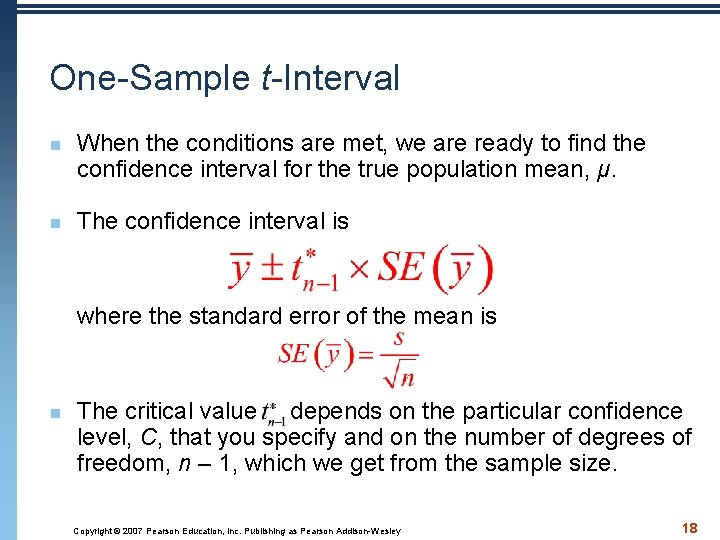One-Sample t-Interval n n When the conditions are met, we are ready to find the confidence interval for the true population mean, μ. The confidence interval is where the standard error of the mean is n The critical value depends on the particular confidence level, C, that you specify and on the number of degrees of freedom, n – 1, which we get from the sample size. Copyright © 2007 Pearson Education, Inc. Publishing as Pearson Addison-Wesley 18Example: Triphammer Road is a busy street that passes through a residential neighborhood. Residents there are concerned that vehicles traveling on Triphammer often exceed the posted speed limit of 30 miles per hour. The local police sometimes place a radar speed detector by the side of the road; as a vehicle approaches, this detector displays the vehicle’s speed to its driver. The police hope that drivers will slow down if they are reminded of their speed. The local residents are not convinced that such a passive method is helping the problem. They wish to persuade the village to add extra police patrols. To help their case, a resident stood where he could see the detector and recorded the speed of vehicles passing it during a 15 minute period on day. The speeds of the vehicles are given. Let’s build a 90% confidence interval for the mean speed of all vehicles traveling on Triphammer Road. Copyright © 2007 Pearson Education, Inc. Publishing as Pearson Addison-Wesley 19Example 1 continued…. I wish to find a 90% confidence interval for the mean speed, μ, of vehicles driving on Triphammer Road. Model: 1. Independence: Cars are independent of each other. 2. Randomization: Not really met. This is a convenience sample, but I have reason to believe that it is a representative sample. 3. 10% condition: The 23 cars observed in the sample is fewer than 10% of all cars that travel on this road. 4. Nearly Normal Condition: Since n<30, we will graph the data. Since the data is unimodal and approximately symmetric, the sampling distribution model is nearly Normal. YOU MUST DRAW BOXPLOT – labeled and to scale Since the conditions are satisfied, I will find a one-sample t-interval for the mean using 22 degrees of freedom. Copyright © 2007 Pearson Education, Inc. Publishing as Pearson Addison-Wesley 20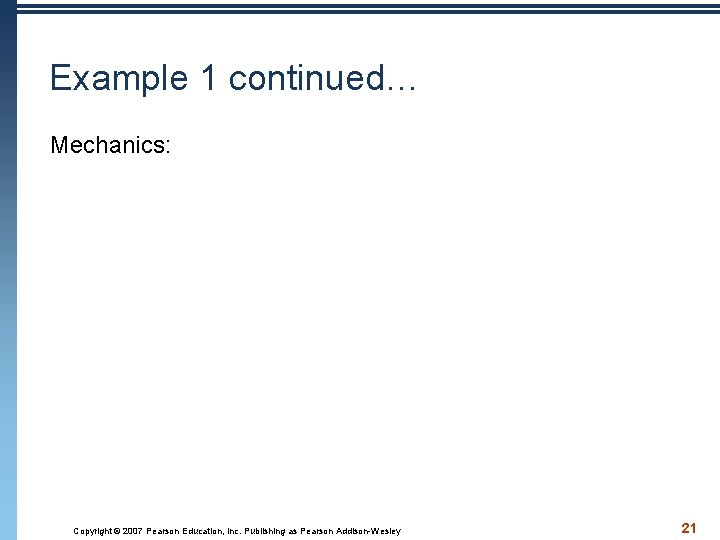Example 1 continued… Mechanics: 1. Enter data into L 1. Since you have already created the boxplot, it should be there now. 2. Choose TInterval under Stat Tests 3. Choose: Inpt: Data and specify your data is L 1. 4. Choose the confidence level you want: 90 5. Calculate 6. Write down: your interval, mean, standard deviation. Copyright © 2007 Pearson Education, Inc. Publishing as Pearson Addison-Wesley 22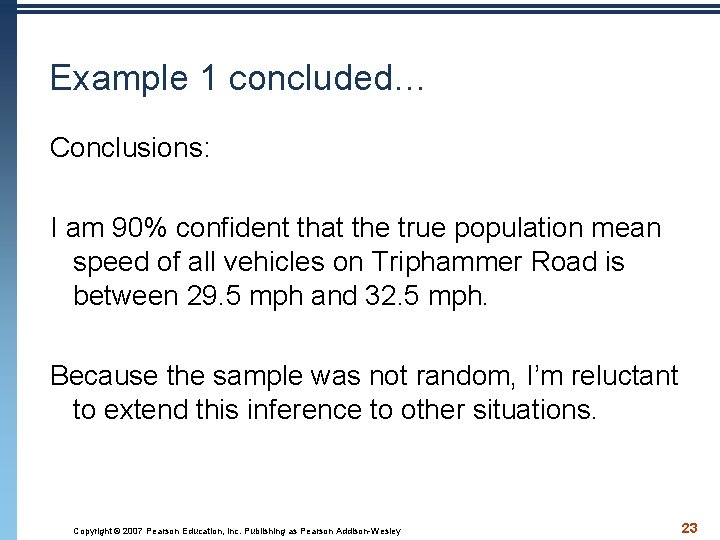Example 1 concluded… Conclusions: I am 90% confident that the true population mean speed of all vehicles on Triphammer Road is between 29. 5 mph and 32. 5 mph. Because the sample was not random, I’m reluctant to extend this inference to other situations. Copyright © 2007 Pearson Education, Inc. Publishing as Pearson Addison-Wesley 23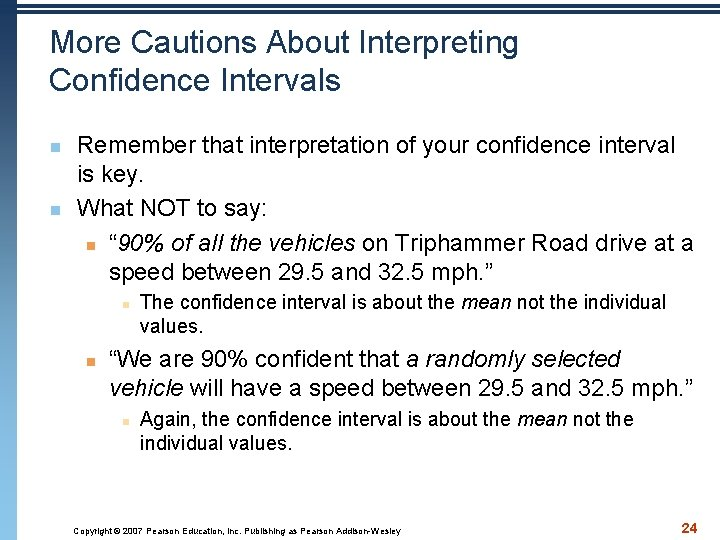More Cautions About Interpreting Confidence Intervals n n Remember that interpretation of your confidence interval is key. What NOT to say: n “ 90% of all the vehicles on Triphammer Road drive at a speed between 29. 5 and 32. 5 mph. ” n n The confidence interval is about the mean not the individual values. “We are 90% confident that a randomly selected vehicle will have a speed between 29. 5 and 32. 5 mph. ” n Again, the confidence interval is about the mean not the individual values. Copyright © 2007 Pearson Education, Inc. Publishing as Pearson Addison-Wesley 24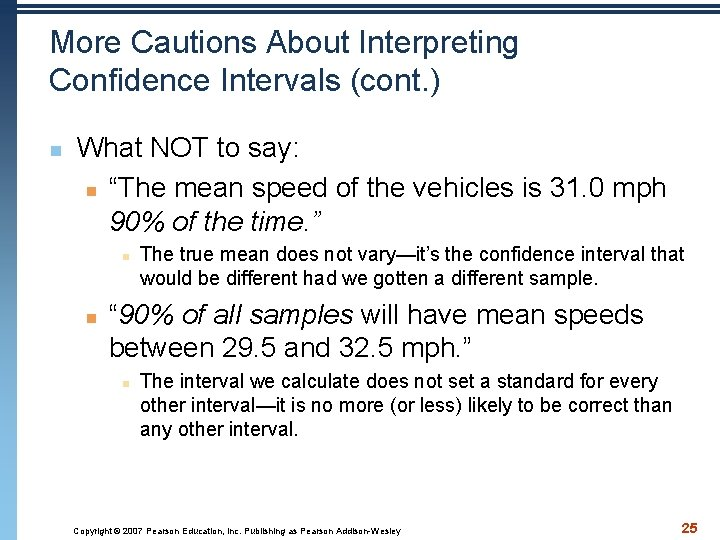More Cautions About Interpreting Confidence Intervals (cont. ) n What NOT to say: n “The mean speed of the vehicles is 31. 0 mph 90% of the time. ” n n The true mean does not vary—it’s the confidence interval that would be different had we gotten a different sample. “ 90% of all samples will have mean speeds between 29. 5 and 32. 5 mph. ” n The interval we calculate does not set a standard for every other interval—it is no more (or less) likely to be correct than any other interval. Copyright © 2007 Pearson Education, Inc. Publishing as Pearson Addison-Wesley 25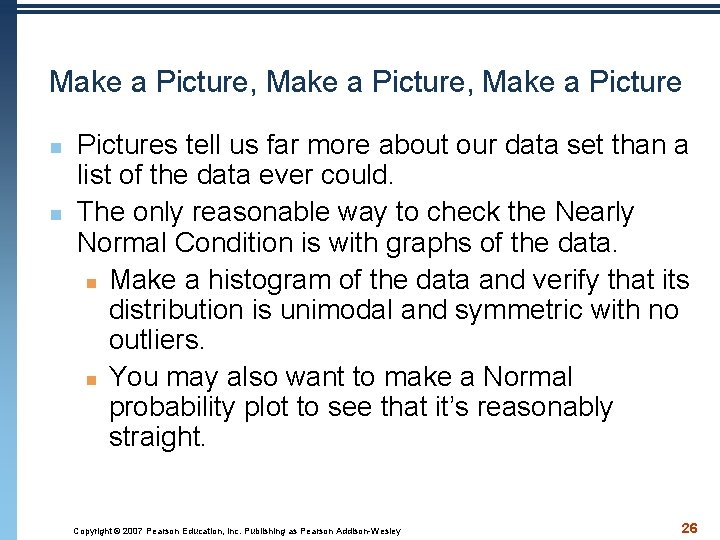Make a Picture, Make a Picture n n Pictures tell us far more about our data set than a list of the data ever could. The only reasonable way to check the Nearly Normal Condition is with graphs of the data. n Make a histogram of the data and verify that its distribution is unimodal and symmetric with no outliers. n You may also want to make a Normal probability plot to see that it’s reasonably straight. Copyright © 2007 Pearson Education, Inc. Publishing as Pearson Addison-Wesley 26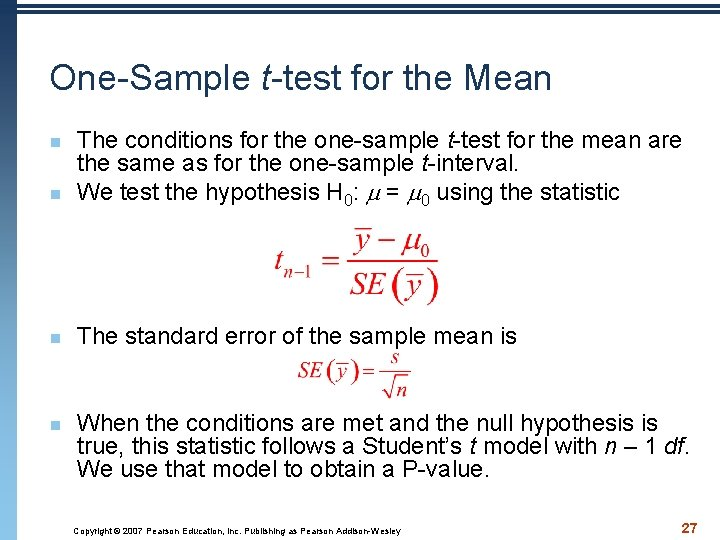One-Sample t-test for the Mean n The conditions for the one-sample t-test for the mean are the same as for the one-sample t-interval. We test the hypothesis H 0: = 0 using the statistic n The standard error of the sample mean is n n When the conditions are met and the null hypothesis is true, this statistic follows a Student’s t model with n – 1 df. We use that model to obtain a P-value. Copyright © 2007 Pearson Education, Inc. Publishing as Pearson Addison-Wesley 27Significance and Importance n Remember that “statistically significant” does not mean “actually important” or “meaningful. ” n Because of this, it’s always a good idea when we test a hypothesis to check the confidence interval and think about likely values for the mean. Copyright © 2007 Pearson Education, Inc. Publishing as Pearson Addison-Wesley 28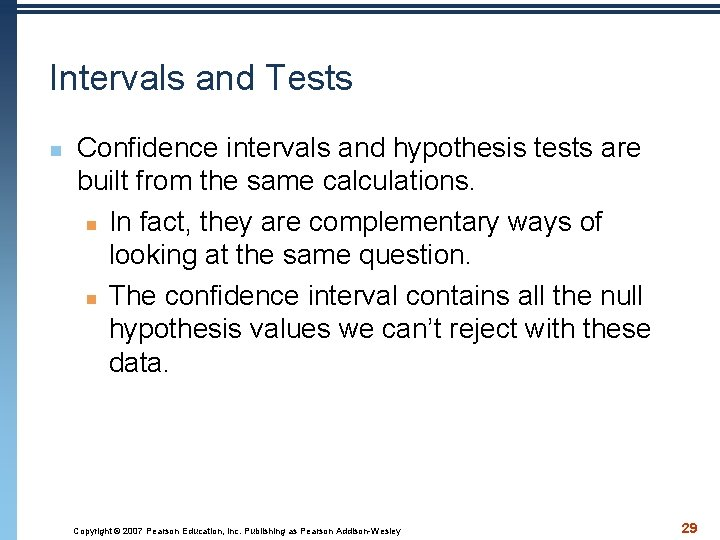Intervals and Tests n Confidence intervals and hypothesis tests are built from the same calculations. n In fact, they are complementary ways of looking at the same question. n The confidence interval contains all the null hypothesis values we can’t reject with these data. Copyright © 2007 Pearson Education, Inc. Publishing as Pearson Addison-Wesley 29Intervals and Tests (cont. ) n n More precisely, a level C confidence interval contains all of the possible null hypothesis values that would not be rejected by a two-sided hypothesis text at alpha level 1 – C. n So a 95% confidence interval matches a 0. 05 level test for these data. Confidence intervals are naturally two-sided, so they match exactly with two-sided hypothesis tests. n When the hypothesis is one sided, the corresponding alpha level is (1 – C)/2. Copyright © 2007 Pearson Education, Inc. Publishing as Pearson Addison-Wesley 30Example: Coffee n A coffee machine dispenses coffee into paper cups. You’re supposed to get 10 ounces of coffee, but the amount varies slightly from cup to cup. Here are the amounts measured in a random sample of 20 cups. Is there evidence that the machine is shortchanging customers? Copyright © 2007 Pearson Education, Inc. Publishing as Pearson Addison-Wesley 31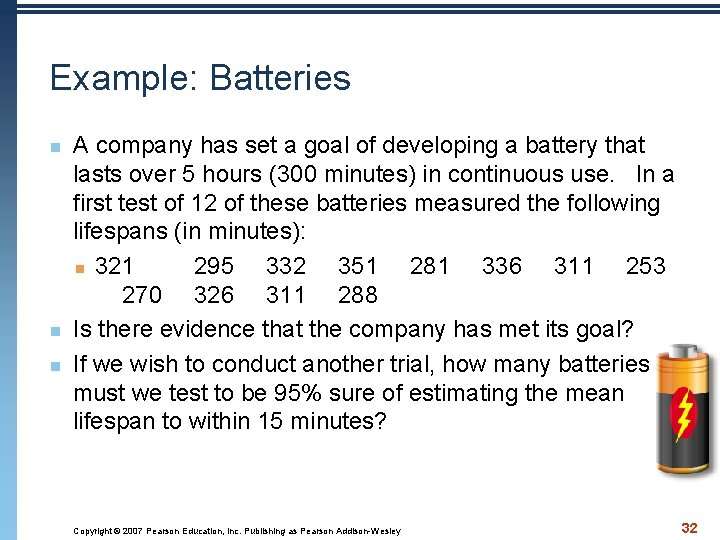Example: Batteries n n n A company has set a goal of developing a battery that lasts over 5 hours (300 minutes) in continuous use. In a first test of 12 of these batteries measured the following lifespans (in minutes): n 321 295 332 351 281 336 311 253 270 326 311 288 Is there evidence that the company has met its goal? If we wish to conduct another trial, how many batteries must we test to be 95% sure of estimating the mean lifespan to within 15 minutes? Copyright © 2007 Pearson Education, Inc. Publishing as Pearson Addison-Wesley 32Sample Size n n To find the sample size needed for a particular confidence level with a particular margin of error (ME), solve this equation for n: The problem with using the equation above is that we don’t know most of the values. We can overcome this: n We can use s from a small pilot study. n We can use z* in place of the necessary t value. Copyright © 2007 Pearson Education, Inc. Publishing as Pearson Addison-Wesley 33Sample Size (cont. ) n n n Sample size calculations are never exact. n The margin of error you find after collecting the data won’t match exactly the one you used to find n. The sample size formula depends on quantities you won’t have until you collect the data, but using it is an important first step. Before you collect data, it’s always a good idea to know whether the sample size is large enough to give you a good chance of being able to tell you what you want to know. Copyright © 2007 Pearson Education, Inc. Publishing as Pearson Addison-Wesley 34*The Sign Test—Back to Yes and No n n We could turn our quantitative data into a set of yes/no values (Bernoulli trials). We can test a median by counting the number of values above and below that value—this is called a sign test. n The sign test is a distribution-free method, since there are no distributional assumptions or conditions on the data. n Because we no longer have quantitative data, we don’t require the Nearly Normal Condition. Copyright © 2007 Pearson Education, Inc. Publishing as Pearson Addison-Wesley 35What Can Go Wrong? Ways to Not Be Normal: n Beware multimodality. n The Nearly Normal Condition clearly fails if a histogram of the data has two or more modes. n Beware skewed data. n If the data are very skewed, try re-expressing the variable. n Set outliers aside—but remember to report on these outliers individually. Copyright © 2007 Pearson Education, Inc. Publishing as Pearson Addison-Wesley 36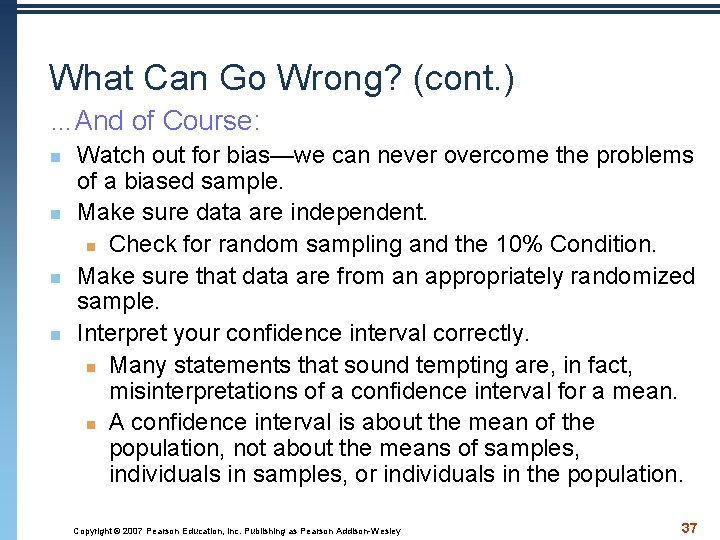What Can Go Wrong? (cont. ) …And of Course: n Watch out for bias—we can never overcome the problems of a biased sample. n Make sure data are independent. n Check for random sampling and the 10% Condition. n Make sure that data are from an appropriately randomized sample. n Interpret your confidence interval correctly. n Many statements that sound tempting are, in fact, misinterpretations of a confidence interval for a mean. n A confidence interval is about the mean of the population, not about the means of samples, individuals in samples, or individuals in the population. Copyright © 2007 Pearson Education, Inc. Publishing as Pearson Addison-Wesley 37What have we learned? n n Statistical inference for means relies on the same concepts as for proportions—only the mechanics and the model have changed. The reasoning of inference, the need to verify that the appropriate assumptions are met, and the proper interpretation of confidence intervals and P-values all remain the same regardless of whether we are investigating means or proportions. Copyright © 2007 Pearson Education, Inc. Publishing as Pearson Addison-Wesley 38